American Journal of Aerospace Engineering
Volume 3, Issue 1-1, February 2016, Pages: 17-21

Natural Convection in an Anisotropic Non-Darcy in Differentially Heated Porous Cavity Under G-Jitter

P. Ghosh*, S. Tuteja

Department of Mechanical Engineering Indian Institute of Technology(Banaras Hindu University), Varanasi, Uttar Pradesh, India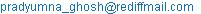(P. Ghosh)

P. Ghosh, S. Tuteja. Natural Convection in an Anisotropic Non-Darcy in Differentially Heated Porous Cavity Under G-Jitter. American Journal of Aerospace Engineering. Special Issue: Space Laboratories: History, Researches, Prospects. Vol. 3, No. 1-1, 2015, pp. 17-21. doi: 10.11648/j.ajae.s.2016030101.14

Abstract: Natural convection in a square porous cavity under sinusoidal g-jitter has been studied for hydro dynamically and thermally anisotropic porous media. The difference with the homogeneous porous media under sinusoidal g-jitter with the anisotropic porous medium under sinusoidal g-jitter is the circulation pattern change. Fluid flow aligns with the porosity distribution. An effort has also been made to understand the non-Darcy effect for the above mentioned problem. It has been observed that at very low velocities, results from the porous media following Darcy’s model and Forchheimer’s equation (non- Darcy model) closely resemble each other. Velocity and pressure behave in a sinusoidal fashion with the same frequency as with the gravitational acceleration. Last but not the least an effort has also been made to understand the behaviors of average Nusselt number in the above mentioned situations.

Keywords: Anisotropic, G-Jitter, Forchheimer’s Equation, Non-Darcy, Porous Media, Sinusoidal

1. Introduction

Microgravity indicates low gravity where the main gravity ranges between 10-1 to 10-5 m/s2. It was considered that space environment was completely weightless. All onboard objects (Space Shuttle and the International Space Station) experience low amplitude broadband perturbed acceleration, or g-jitter, where there is a non-zero steady (metastable) part and an oscillatory part (random and constructive) [1,2]. This perturbed residual acceleration is called "g-jitter. Experiments have been carried out in space, to find out the influence of the g-jitter.

Porous media is used in many areas of applied science and engineering: filtration, mechanics (soil mechanics, rock mechanics), engineering (petroleum engineering, construction engineering), geosciences (hydrogeology, petroleum geology, geophysics), biology and biophysics, material science, etc. Natural convection through porous media under g jitter has emerged as a subject of interest with interesting applications biological and material science.

Thermal convection in a layer heated from below for the study of non-linear fluid dynamics and the transition to turbulence have been studied by Busse (1978) . Natural convection in porous medium heated from below was investigated by Cheng (1978) . Biringen and Danabasoglu (1990)  solved fully nonlinear time-dependent Boussinesq equations for g-jitter in a two-dimensional rectangular cavity with an aspect ratio of 2 at Ra = 1.775×105 and at a Prandtl number (Pr) = 0.007 (liquid germanium). They specified the critical value of ω above which the system experiences transition from convective temperature fields to a conductive one.

A detailed flow field and heat transfer analysis under g-jitter with a zero-mean base gravity parallel to the applied thermal gradient inside the enclosure has been studied by Hirata et al. (2001)  which also includes the analysis of onset of convection. Natural convection in an isotropic Darcy square porous cavity under constant gravity has been studied. The result is validated with a similar study conducted by Ghosh and Ghosh  .

However, there isn’t any experimental or computational study for natural convection in a anisotropic square cavity where g-jitter is applied perpendicular to the thermal gradient. Moreover, Natural convection in a Non-Darcy medium under g-jitter has not been studied. In many applications, porous materials are anisotropic due to preferential orientation for solid bundles. A detailed analysis on natural convection in a heat generating anisotropic porous medium is yet to appear in the literature. The paper deals with the study of flow behaviour in isotropic and anisotropic porous medium in which fluid is subjected to g-jitter applied in a particular direction. Further, Non Darcy model has to be applied to the porous medium, and its effect has to be studied. Moreover an effort has also been made in a Non Darcy porous medium following Forchheimer’s equation has also been analysed.

2. Transport Equations and Numerical Solution Methodology

A two-dimensional square cavity has been modelled and hexahedral mesh is generated for discretization of the domain. Figure 1 shows the schematic diagram of the domain to be analysed. G-jitter is applied parallel to Y-axis. Top and bottom walls parallel to X-axis are adiabatic. Left and right walls are isothermal with different temperatures applied to them. It is assumed that isothermal walls are perfect conductors of heat and that the square cavity is completely filled with homogeneous porous media saturated with Newtonian fluid. Different temperatures at the walls create density differences in the fluid which produces buoyancy and drives convection. Number of computational cells has been kept at 2500 after performing the grid independence tests.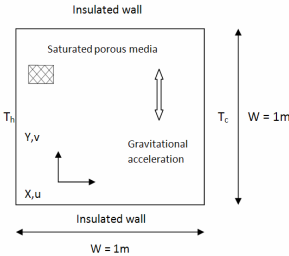Figure 1. The CFD domain.

In a porous medium following the Darcy model, the x directional pore velocity (u) and y directional pore velocity (v) can be presented as follows,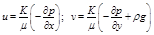Using Darcy’s law the dimensionless transport equations under sinusoidal g-jitter are as follows. Heat transfer through the porous medium is represented, subject to the assumption of thermal equilibrium between the medium and the fluid flow, by the energy equation below.

All asterisked quantities and θ in this paper are in dimensionless form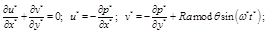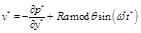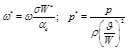Where,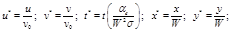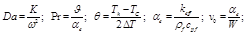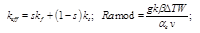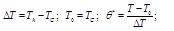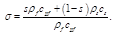TheNon-Darcy porous layer follows Forchheimer’s equation. According to Joseph et al. (1982)  the appropriate modification to Darcy’s equation is to replace it by: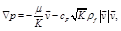where cF is a dimensionless form-drag constant.

This is the Forchheimer’s equation and the last term is call the forchheimer’s term. Using Forchheimer’s equation the dimensionless transport equations under sinusoidal g-jitter are as follows.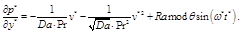The energy equation remains the same as in the Darcy model: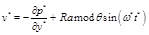Air and aluminium has been used as the fluid and porous solid respectively. Incompressible ideal gas model is used for varying air density. The base microgravity (10−6g0) [1,2] has been determined by solving transport equations numerically.

The source term added to the y direction momentum equation is shown below: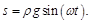An unsteady segregated solver is used and second order implicit time marching scheme is used. Second order upwind scheme is used for momentum and energy discretization. The Body Force- Weighted scheme is applied for pressure discretization. The SIMPLE algorithm is applied as a standard scheme for pressure- velocity coupling. Fixed time stepping method is used for time marching. Residual normalization method is used for reaching the convergence criterion.

3. Isotropic Darcy Square Porous Cavity Under Constant Gravity

Natural convection in an isotropic Darcy square porous cavity under constant gravity has been studied. The result is validated with a similar study conducted by Ghosh and Ghosh (2009) . Results are presented for Ramod = 100. Fluid circulation is observed and the velocity contour plot is shown in the figure 2.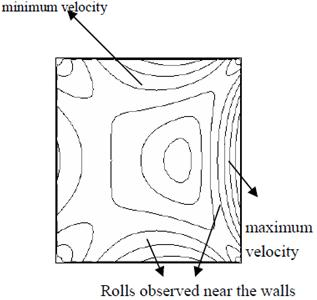Figure 2. Velocity contours in porous cavity under constant gravity.

The centre of rotation is near the cold wall towards the right of the cavity centre. Greater convection effects are seen near the cold wall resulting in greater velocities. Rolls are observed near the walls. These rolls are due to the thermal diffusivity difference of wall material, fluid and solid matrix.

The maximum and minimum velocities of 3.41·10-9 m/s and 4.26·10-10 m/s are observed in the entire porous cavity. In the mid plane of the cavity at the upper half, flow is from hot side to cold wall and in the lower half the flow is from cold side to hot wall, which clearly indicate a clockwise rotation.

Table 1 and fugure 3 shows the average Nusselt numbers calculated in the present study and in the study conducted by Ghosh and Ghosh (2009) .

Table 1. Comparison of average Nusselt number with numerical results by Ghosh and Ghosh (2009) at constant gravity (sin(ωt) =1).

 Name Ramod = 100 Ramod = 1000 Present prediction 3 13,2 Ghosh and Ghosh (2009) 3,14 13,80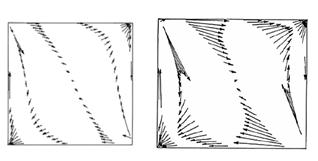Figure 3. Velocity vectors in porous cavity under constant microgravity (10mm/s2) in present model (left) and simulations (right) performed by Ghosh and Ghosh (2009) .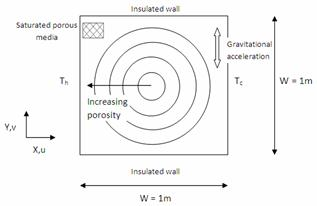Figure 4. Anisotropic porous medium with five different porosity values in different concentric circular regions.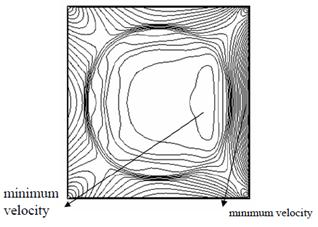Figure 5. Velocity contour in the anisotropic porous cavity under constant gravity.

4. Anisotropic Darcy Porous Layer Under G-Jitter

Natural convection in the anisotropic(different porosity in different circular region) square porous cavity under g-jitter for Ramod =100, and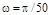has been studied. Gravity peaks at every 25 s and reverses its direction every 50 s. Figure 4 shows the anisotropic porous medium with five different porosity values in different concentric circular regions. Porosity increases from 0.6 in the inner circle to 0.8 towards the walls. Figure 5 shows the velocity contours for natural convection in the anisotropic porous medium under constant gravity.

Fig. 6 show velocity vectors at Ramod = 100 andat different instances of time.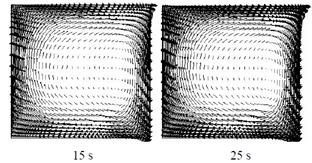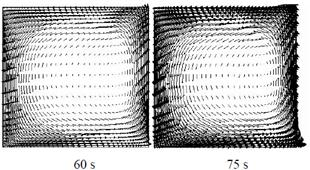Figure 6. Vectors in the anisotropic porous cavity for Ramod = 100, ω=π/50.

The maximum and minimum velocities are 6.2·10-9 m/s and 5.2 ·10-10 m/s. The flow field is divided in zones of different velocities. The region with maximum porosity has maximum velocity. Though the centre has the lowest porosity, it does not have the lowest velocity. The region with the minimum velocity is towards the right of the centre near the cold wall. The highest velocity is seen near the cold wall.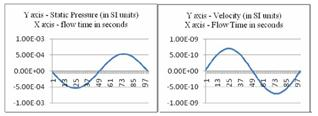Figure 7. Static Pressure (N/m2) and velocity (m/s) at point (0.8, 0.4).

Under g-jitter fluid behaviour is periodic with the same time period as that of the gravitational acceleration. The velocity and pressure behaviour is same for all points in the porous media. Fluid circulation changes from clockwise to anticlockwise direction at t=50 s and fluid velocity peaks at 25 s and 75 s. Fig 7 shows that the fluid velocity and pressure at point 3 behave in a sinusoidal manner.

The temperature remains constant at a point in the porous media at all instances of time. The magnitudes of the average Nusselt number at the hot wall remains constant with time. Table 2 shows the magnitude of average Nusselt number in the anisotropic porous medium. The average Nusselt number in the case with circular porosity function is greater than in earlier discussed cases. This indicates that convection effect is more dominant than that in the earlier discussed cases.

Table 2. Average Nusselt number for oscillating gravity, ω=π/50.

 Parametr Ramod = 100 Ramod = 1000 Average Nu 3,6 14,1

5. Isotropic Non-Darcy Square Porous Cavity Under G-Jitter

In this case, the isotropic porous medium follows the Forchheimer’s equation. As observed from Fig 8, the velocity contour is similar to that in the isotropic Darcy case.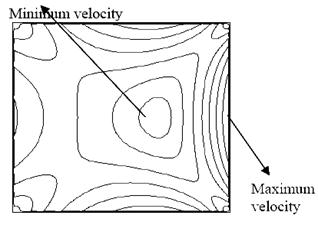Figure 8. Velocity contour in the Non-Darcy porous cavity under constant gravity.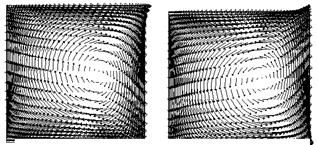Figure 9. Velocity vectors in the Non-Darcy porous cavity for Ramod = 100, ω=π/50.

The maximum and minimum velocities are 3.41·10-9 m/s and 7.9·10-11 m/s which are comparable with those in the Darcy case. There is no significant difference between the Darcy and Non-Darcy cases. Fluid behaviour is periodic with the same time period as that of the gravitational acceleration. Fluid circulation changes from clockwise to anticlockwise direction at t=50 s and fluid velocity peaks at 25 s and 75 s (fugure 9).

Under g-jitter, the temperature remains constant at a point in the porous media at all instances of time. The magnitudes of the average Nusselt number at the hot wall remains constant with time. Table 3 shows the magnitude of average Nusselt number in the Non Darcy porous medium. Average Nusselt number magnitude indicates that convection effect is more dominant than that in the earlier discussed cases. This is due to the extra convective term added in the momentum equation.

Table 3. Average Nusselt number for oscillating gravity, ω=π/50.

 Parametr Ramod = 100 Ramod = 1000 Average Nu 3,5 14,3

6. Conclusion

Natural convection in anisotropic porous media under g-jitter has been studied for four different cases. In all the cases, fluid circulation is seen with different centers of circulation as described in the results. Velocity and pressure behave in a sinusoidal fashion with the same frequency as with the gravitational acceleration. The average Nusselt number remains constant at all instances of time under g-jitter. At the hot wall, anisotropic medium with a circular porosity function has a higher average Nusselt number magnitude, while anisotropic medium with porosity increasing in the x directions has a lower average Nusselt number magnitude as compared to the isotropic medium. The average Nusselt number at the hot wall in the Non Darcy porous layer is more than in the Darcy porous layer. This is due to the inertial term in the Forcheimer equation which is not present in the Darcy model equation. Natural convection in an isotropic Non-Darcy porous medium under g-jitter closely resembles that in the Darcy porous medium.

References

1. A.V. Sedelnikov "Classification of microaccelerations according to methods of their control," Microgravity Scienes and Technology, vol. 27, No 3, 2015, pp.327–334.
2. A.V. Sedelnikov "The usage of fractal quality for microacceleration data recovery and for measuring equipment efficiency check," Microgravity Scienes and Technology, vol. 26, No 5, 2014, pp.327–334.
3. F.H. Busse "Non-linear properties of thermal convection," Rep. Prog. Phys., No 41, 1978, pp. 1929-1967.
4. P. Cheng "Heat transfer in geothermal systems," Adv. Heat Transfer, No 14, 1978, pp. 1-105.
5. S. Biringen and G. Danabasoglu "Computation of convective flow with gravity modulation in rectangular cavities," J. Thermophys, No 4, 1990, pp. 357-365.
6. K. Hirata, T. Sasaki and H. Tanigawa "Vibrational effects on convection in a square cavity at zero gravity," J. Fluid Mech., vol. 45, No 4, 2001, pp. 327-344.
7. P. Ghosh and M.K. Ghosh "Streaming flows in differentially heated square porous cavity under sinusoidal g-jitter,"Int. J. Therm. Sc., No 48, 2009, 514-520.
8. D.D. Joseph, D.A. Nield and G. Papanicolaou "Nonlinear equation governing flow in a saturated porous medium," Water Resour. Res., vol. 18, No 4, 1982, pp. 1049-1052.

 Contents 1. 2. 3. 4. 5. 6.
Article ToolsAbstractPDF(459K)